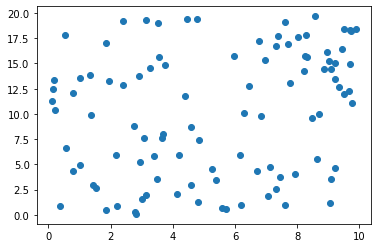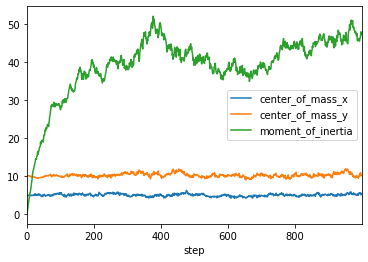# A toy model¶

Let's run a random walk (a bunch of particles moving randomly) in a periodic 2-dimensional domain (a torus), and let's diagnose their center of mass (mean of all positions) and moment of inertia (variance of all positions) after each move.

## Tech preamble¶

In :
%matplotlib inline
import numpy as np
from matplotlib import pyplot as plt
import pandas as pd


## A typical piece of scientific software¶

Typical scientific software looks like this: There's a piece of code that does it all. There are hardly any abstractions. Dependencies are hard to figure out, etc.

See for example Johanson and Hasselbring (2018) for an overview of the state of scientific software.

In :
def run_random_walk(
length_x=10,
length_y=20,
number_particles=100,
number_steps=100,
step_length=0.5
):
# initialize particle positions
x = np.full((number_particles, ), length_x / 2)
y = np.full((number_particles, ), length_y / 2)

# initialize diagnostics
center_of_mass_x = np.empty((number_steps, ))
center_of_mass_x = x.mean()

center_of_mass_y = np.empty((number_steps, ))
center_of_mass_y = y.mean()

moment_of_inertia = np.empty((number_steps, ))
moment_of_inertia = x.var() + y.var()

# main loop
for step in range(number_steps - 1):
x += step_length * (
np.random.normal(size=(number_particles, ))
)
x = np.mod(x, length_x)

y += step_length * (
np.random.normal(size=(number_particles, ))
)
y = np.mod(y, length_y)

center_of_mass_x[step+1] = x.mean()
center_of_mass_y[step+1] = y.mean()
moment_of_inertia[step+1] = x.var() + y.var()

return center_of_mass_x, center_of_mass_y, moment_of_inertia, x, y


But the typical user of scientific software doesn't look at this anyway. They see:

In :
(
center_of_mass_x, center_of_mass_y,
moment_of_inertia,
x, y
) = run_random_walk(number_steps=1000)


## Data and visualizations¶

And then, they look at data:

In :
plt.scatter(x, y);In :
data = pd.DataFrame()
data["center_of_mass_x"] = center_of_mass_x
data["center_of_mass_y"] = center_of_mass_y
data["moment_of_inertia"] = moment_of_inertia
data["step"] = np.arange(len(data))
data.set_index("step")

Out:
center_of_mass_x center_of_mass_y moment_of_inertia
step
0 5.000000 10.000000 0.000000
1 4.976764 10.077956 0.448184
2 4.906573 10.055832 0.950142
3 4.917229 10.096890 1.571212
4 4.873878 10.093618 2.276138
... ... ... ...
995 5.552990 10.882198 46.604325
996 5.250382 10.810701 47.134947
997 5.060288 10.505201 48.048083
998 5.264641 10.283856 47.273895
999 5.378520 10.127725 47.361438

1000 rows × 3 columns

In :
data.plot(x="step");